# Little Circuit Challenge, DC Current In Inductor

#### MrAl

Joined Jun 17, 2014
7,800
Hello there,

Here is what i think is an interesting question. The circuit is shown in the diagram.

The question is, what is the DC current in L2 when the battery voltage has been applied for a long long time (theoretically this would be for time approaching positive infinity).

This is similar to finding the initial current in an inductor when it was initialized with a battery like that and there is another time varying source.

This is not a trick question or anything, but i think there may be two or more answers that would be reasonable. It's mainly for the purpose of starting a discussion on this.
If you decided on an answer (or two or more) please state why you think it is so.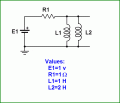#### OBW0549

Joined Mar 2, 2015
3,545
The question is, what is the DC current in L2 when the battery voltage has been applied for a long long time (theoretically this would be for time approaching positive infinity).
Assuming L1 and L2 are ideal (i.e., they have no intrinsic resistance) my guess would be 1/3 of an ampere.

My reasoning is that from the time the voltage source E1 is applied onward, at all times the voltage across L1 and L2 will cause twice the dI/dT in L1 as is caused in L2, since L2 has twice the inductance of L1. Integrated over time, this leads to L1 carrying twice the current carried by L2. Since the total current is 1 ampere (E1/R1), the current through L2 is 1/3 ampere.

I think...

Last edited:
•sagor

#### Kjeldgaard

Joined Apr 7, 2016
410
With the assumption that coils and wires are superconducting, my bid is that the two coils share the one ampere current.

So the current in L2 will be half an ampere.

#### mvas

Joined Jun 19, 2017
537
It depends upon the DC Resistance ( DCR ) of the two inductors - L1 & L2.

The two inductors are in parallel and then they form a simple voltage divider with R1 ...
L2 (amps) = E1 * ( X / ( X + R1 ) ) / DCRL2

Where X is the Parallel DC Resistance of L1 & L2
X = ( DCRL1 * DCRL2 ) / ( DCRL1 + DCLR2 )
DCRL1 = DC Resistance of L1
DCRL2 = DC Resistance of L2

You did not specify the DC Resistance of L1 or L2, therefore the final answer is unknown until you do.
The current flowing through L2 is greater than 0 amps and less than 1 amp, until more details are provided.

Last edited:

#### AlbertHall

Joined Jun 4, 2014
10,388
Assuming L1 and L2 are ideal
And assuming their magnetic fields do not interact with each other or their surroundings and that the core material does not saturate then I agree - 1/3A.

•sagor

#### MrAl

Joined Jun 17, 2014
7,800
Hi again,

The reason i brought this up was in another thread the question included two inductors in parallel and the member did not know what to do. I can see why cant you. We need more specification.

For one, if the inductors were both wound with the same wire inductor 2 would have more resistance so that would affect it.

But in theory, if we do a step response we get a ratio of inductances only (with zero resistances).
On the other hand, if we think of it as real DC then we get equal current in both inductors.
So there is a paradox here in theory. That i think applies to the other thread question (in Homework).

#### The Electrician

Joined Oct 9, 2007
2,784
But in theory, if we do a step response we get a ratio of inductances only (with zero resistances).
On the other hand, if we think of it as real DC then we get equal current in both inductors.
Could you explain with more detail what you mean by "real DC"? I'm guessing you mean a voltage that doesn't turn on in a step function, but rather has existed since t = -∞. Whatever it is, why would it result in equal currents in both inductors?

I think we can learn a lot by setting up differential equations for your circuit and solving various cases.

I chose to do a loop analysis with two loop currents I1 and I2. For now, the inductors are assumed to have zero resistance. The I1 loop traverses E1, R1 and L1. The I2 loop traverses E1, R1 and L2. With these definitions the branch current in L1 is the same as the loop current I1, and the branch current in L2 is the same as loop current I2: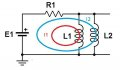If we let the currents in L1 and L2 be our initial conditions we can solve several cases. E1 is treated as a step of 1 volt at t=0. We'll solve for the two loop currents as functions of time: I1[t] (Blue) and I2[t] (Red). With the initial currents I(L1) and I(L2) set to zero (I1 = 0 and I2 = 0), we get this result:The final currents are 2/3 amps in L1 and 1/3 amp in L2.

If we choose the case where I1 = 1/2 amp and I2 = 1/2 amp, we get this result: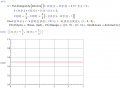There is no time constant involved; the currents are both 1/2 amp at time t=0 right away. The red and blue current curves in the image are superimposed horizontal lines at 1/2 amp. Perhaps this is the case you are alluding to.

But, there's no reason why the initial currents have to be 1/2 amp. In fact, if the two initial currents are any two values that add up to exactly 1 amp, there is no time constant involved, and the currents remain at their initial values for all time. For example, here is the result if we set I1 = 2/7 and I2 = 5/7: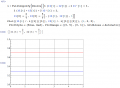•Danko

#### The Electrician

Joined Oct 9, 2007
2,784
The final currents are not necessarily in the ratio of L2/L1 (or its inverse). For the case where the sum of the initial currents is exactly 1, the ratio of the currents is unrelated to the values of L1 and L2. If the initial currents don't sum to 1, the behavior has a time constant, but the final currents are not necessarily in the ratio L2/L1 (or its inverse). For example, let I1 = 2/10 and I2 = 1. The result is: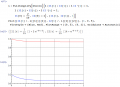Finally, if we allow the inductors to have some non-zero resistance, we would expect the final (t >>> 0) currents to be just as a DC analysis would predict. Here's the result when each inductor has a resistance 0f .02 ohms and the initial currents are zero as in the very first image in the previous post. The plot extends to 100 seconds so we can see that the currents are asymptotically approaching .48 amp in each inductor after the faster initial approach to currents of 2/3 amp in L1 and 1/3 amp in L2: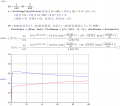Last edited:

#### BR-549

Joined Sep 22, 2013
4,938
I guess I don't understand the setup premise and how it relate to the question. Very confusing....the circuit response to turn on time is extreme. I would think the only thing limiting current after a very short time.....would be R1, in series with the parallel R of the coils.

1H and 2H coils have resistance. Much more than 1 ohm.

If the inductors are ideal.....then 1/2 amp.

But everyone knows this. The coil is impotent without change.

#### Sensacell

Joined Jun 19, 2012
2,595
The final coil current is determined by the unspecified coil resistance.

No answer can be determined based on the provided information.

•sagor

#### The Electrician

Joined Oct 9, 2007
2,784
The final coil current is determined by the unspecified coil resistance.

No answer can be determined based on the provided information.
Other posters have also suggested that the currents cannot be determined without knowing the inductor resistances. Suppose we let the inductors have a very small resistance, say 1 milliohm. For inductors of 1 H and 2H, 1 milliohm is quite small. Since the time constant of an inductor plus resistance is given by L/R, the time constant of inductors of 1 and 2 henries with resistance of 1 milliohm would be around 1000 seconds. This would mean that for 5 seconds we shouldn't see much effect due to the resistance, but including some finite resistance would satisfy those who believe the final current can't be determined unless the inductors have some resistance. So let's solve the differential equations with 1 milliohm resistance for each inductor and plot the result for several time intervals.

First, using the loop currents I described in post #7, here is the solution plotted for the first 5 seconds after application of the 1 volt step, with the initial currents in the inductors set to zero. Compare this to the first image in post #7 where the inductors were ideal, with no resistance: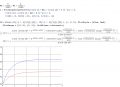Here is the solution plotted for the first 100 seconds: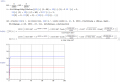#### The Electrician

Joined Oct 9, 2007
2,784
Here is the solution plotted for the first 1000 seconds: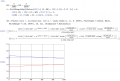And, finally, the solution plotted for the first 10000 seconds: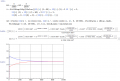For the first 5 seconds, the result is essentially indistinguishable from the first image in post #7, the zero resistance case. For the 10000 second time interval, we see that the final currents are going to be about 1/2 amp in each inductor, just what we would expect due to the resistances of the inductor.

Now, just imagine that the resistance of each inductor is reduced by a factor of 10. What would one expect the plot just above here to look like? It would look the same, except the approach of the currents to 1/2 amp each would take 10 times as long. What if the resistances are 1 microohm? One nanoohm? One picoohm? Is there any reason to think that the first 5 seconds would be noticeably different for these cases of decreasing inductor resistance?

Considering the behavior of these cases of smaller and smaller inductor resistance leads one to believe that the first image in post #7 is correct--with initial currents of zero and with zero inductor resistances, the currents exponentially approach values of 2/3 amps for L1 and 1/3 amp for L2, and remain at those values for t >>> 0.

Clearly, it is possible to calculate the currents with zero resistance in the inductors, as was done in post #7. All one has to do is solve the differential equations. There is no problem doing this; no indeterminate result occurs; you just have to pick appropriate initial conditions.

#### The Electrician

Joined Oct 9, 2007
2,784
I guess I don't understand the setup premise and how it relate to the question. Very confusing....the circuit response to turn on time is extreme. I would think the only thing limiting current after a very short time.....would be R1, in series with the parallel R of the coils.

1H and 2H coils have resistance. Much more than 1 ohm.

If the inductors are ideal.....then 1/2 amp.
Solving the network equations gives a different result.

But everyone knows this. The coil is impotent without change.
This is a good point. There is change, a voltage is applied at time t=0, and this change is what allows us to calculate the currents.

The point of the thread is to answer the question of whether it is possible to calculate the currents when a voltage is suddenly applied to the circuit, given that the inductors are ideal, with no resistance. I've shown that the answer is yes, it is possible to calculate the currents.

#### MrAl

Joined Jun 17, 2014
7,800
Solving the network equations gives a different result.

This is a good point. There is change, a voltage is applied at time t=0, and this change is what allows us to calculate the currents.

The point of the thread is to answer the question of whether it is possible to calculate the currents when a voltage is suddenly applied to the circuit, given that the inductors are ideal, with no resistance. I've shown that the answer is yes, it is possible to calculate the currents.
Hi,

When you use the step response to calculate the DC currents you do so assuming that there was a step response. Recall that i had calculated the step response too. But is that really the way we determine the DC initial conditions of a circuit with a DC source? No not really. We usually use another method which is simply to short out the inductor (in the case of a single inductor) and calculate the current. So remove L2 for a minute and calculate the initial DC current in L1. It is clearly 1/1=1 amp. Do it again but this time remove L1, the current in L2 is 1 amp. Now it makes sense to think that an actual DC current would be shared between the two as 1/2 if there was no other spec like resistance.
Note that in both cases we could have calculated the step response at t toward positive infinity, but we dont usually do that.

So my question is which way should we do it and i dont think there is a definite answer unless as others have pointed out we have a specification of resistance to go along with the inductor values.

One point though that i think is interesting is that if we were told that there was a step input at some point in the past then i think we would have to do it that way only, and ignore the other way with a pure DC that existed long ago.

Also, what exactly is a pure DC that existed from long ago. That would have had to be determined from the current of 1 amp and the fact that both inductors had the same resistance, bringing us again to 1/2 amp each. The question then is, is this even possible? The answer is i dont think so unless the inductors were forced to have 1/2 amp each, and that would come from specifying that each one had 1/2 amp before the start of the analysis. I think that's the only way because if they were both created and then placed in parallel, they would both experience the same dv/dt no matter how it changed and thus the current would always depend on their inductance.

#### Plamen

Joined Mar 29, 2015
101
With the assumption that coils and wires are superconducting, my bid is that the two coils share the one ampere current.

So the current in L2 will be half an ampere.
Petkan:
Clearly we need the coil resistances to be more specific. We will add their equivalent resistance to the ballast resistor and calculate the total current. Then we will split it inversely proportionally to coil resistances (using the fact that they are under the same voltage)

#### WBahn

Joined Mar 31, 2012
26,141
Hi,

When you use the step response to calculate the DC currents you do so assuming that there was a step response. Recall that i had calculated the step response too. But is that really the way we determine the DC initial conditions of a circuit with a DC source? No not really. We usually use another method which is simply to short out the inductor (in the case of a single inductor) and calculate the current. So remove L2 for a minute and calculate the initial DC current in L1. It is clearly 1/1=1 amp. Do it again but this time remove L1, the current in L2 is 1 amp. Now it makes sense to think that an actual DC current would be shared between the two as 1/2 if there was no other spec like resistance.
Note that in both cases we could have calculated the step response at t toward positive infinity, but we dont usually do that.
Removing L2 to find the DC current in L1 is completely unjustified. You seem to be trying to use a completely invalid version of superposition.

Furthermore, you go from shorting out the inductor to removing it from the circuit (which is open-circuiting it).

Imagine a circuit in which L1 and L2 are in series. What would the result be of removing L2 to find the DC current in L1?

Or have them in series but also have each have a parallel resistor across it, say R1 and R2. To keep things finite, have a resistor Ro in series between the supply voltage Vo and the inductor/resistor combinations. Please show how removing L2 to find the DC current in L1 and then doing the reverse to find the DC current in L1 yields a correct answer.

Let's put some numbers to this. Make Vo = 10 V, R1 = 4 Ω, R2 = 9 Ω, R3 = 1 Ω, L1 = 1 H, L2 = 2H.

According to you, if we remove L1 then the DC current in L2 is 2 A while when we remove L2 the DC current in L1 is 1 A. Yet if we do it the right way, we get that the DC current in both inductors is 10 A.

Even if this did somehow work, your reasoning for why the actual DC current would be shared equally is specious -- IF the inductors were identical and IF the initial conditions had equal currents in each of them, THEN you could make such an argument based on symmetry. But they aren't identical and we don't know whether the initial conditions were the same for each or not. It comes down to it making "sense" only because that's the result you would like to have.

So my question is which way should we do it and i dont think there is a definite answer unless as others have pointed out we have a specification of resistance to go along with the inductor values.
You either have to specify constraints that make the initial conditions immaterial OR specify the needed initial conditions. You are wanting to do neither. You are imposing the constraint that the initial voltage at t = -∞ is E1 but you won't specify the initial currents in the inductors at that time. This is needed since you also don't specify constraints, such as inductor series resistance, that would make that information immaterial. Can't have it both ways.

One point though that i think is interesting is that if we were told that there was a step input at some point in the past then i think we would have to do it that way only, and ignore the other way with a pure DC that existed long ago.

Also, what exactly is a pure DC that existed from long ago. That would have had to be determined from the current of 1 amp and the fact that both inductors had the same resistance, bringing us again to 1/2 amp each. The question then is, is this even possible? The answer is i dont think so unless the inductors were forced to have 1/2 amp each, and that would come from specifying that each one had 1/2 amp before the start of the analysis. I think that's the only way because if they were both created and then placed in parallel, they would both experience the same dv/dt no matter how it changed and thus the current would always depend on their inductance.
You are making a lot of unsupported assertions here. Take a 1 H superconducting coil and get 10 A persistent flowing downward in it. Take another superconducting coil of 2 H and get a 9 A persistent current flowing upward in it. Now connect them in parallel and put them into that circuit with your 1 V, 1 Ω source. At this point you will have 10 A in one coil, 9 A in the other, 1 A in the resistor, and the persistent mode switches will have a zero net current in them. Now open the switches. You will have 10 A flowing downward in the first coil, 9 A flowing upward in the second, and and 1 A flowing in the resistor. Note that the details of whether the DC voltage was somehow "pure" is completely immaterial because the assembly of the circuit is not dependent on that in any way.

#### crutschow

Joined Mar 14, 2008
25,389
For zero coil resistance the currents divide 1/3, 2/3, no matter how or when the original voltage was applied.
If the coil resistances are proportional to the inductance, then the coil currents are still 1/3, 2/3.

#### WBahn

Joined Mar 31, 2012
26,141
For zero coil resistance the currents divide 1/3, 2/3, no matter how or when the original voltage was applied.
Read the post above yours (which you might not have gotten to yet). I gave a specific example where this won't be the case -- a case that can be demonstrated in the real world using superconducting coils.

#### The Electrician

Joined Oct 9, 2007
2,784
For zero coil resistance the currents divide 1/3, 2/3, no matter how or when the original voltage was applied.
For the zero coil resistance case, this is only always true if the initial currents in the inductors are both zero when the voltage is applied. Otherwise, if there are initial currents in the inductors when the voltage is applied, and if the sum of those currents is 1 A, then those currents will persist for all time (as with WBahn's example). If the sum of the initial currents is something other than 1 A, the currents will vary exponentially for a while, and then settle down to values that will persist.